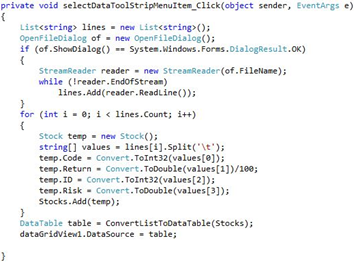### Optimum Portfolio Selection Using a Hybrid Genetic Algorithm and Analytic Hierarchy Process: An Application to Amman Stock Exchange

###### Journal Name : SunText Review of Arts & Social Sciences

Article Type : Research Article

Authors : Al Hallaq ,Ajlouni MM and Al Saadi MF

Keywords : Hybrid genetic algorithm; Analytic hierarchy process; Portfolio selection; Optimum portfolio; Amman stock exchange

### Abstract

The aim of this study is to investigate the ability of a hybrid genetic algorithm (HGA) and analytic hierarchy process (AHP) in selecting the optimum portfolio. This of course, helps investors to decide the most appropriate investment alternatives. For that purpose, the study creates portfolios using daily returns of the companies listed in Amman Stock Exchange, for the period from January 1, 2015 to December 31, 2015. The results show that HGA can identify portfolios that are in the efficient frontier. HGA has advantages over disadvantages for the portfolio selection cases in which the scale of the problem or the nonlinear constraints cannot be solved by linear or quadratic models. In addition, the results reveal that AHP can select the optimum portfolio among the portfolios obtained by HGA.

### Introduction

Selecting investment portfolio is one of the most important research areas in modern finance science; it is seeking to better allocation of funds between baskets of securities. Portfolio selection was introduced first by "Harry Markowitz" in 1952, in his paper "portfolio selection." He explained the concept of diversification, and suggested that investors focus on portfolio selection depending on their overall risk-reward characteristics . Markowitz explained the best way to allocate the money over risky asset in a fixed position. The model of Markowitz consists of only two factors, which are the expected return and variance, and presume investors are risk averse. The idea of the model is that an investor cannot achieve a high return without increasing portfolio risk .

Since Markowitz until today, many attempts been conducted in the portfolio management to find new mathematical approaches to select the optimum portfolio. The computational capacity of the 21st century and the wide availability of computers have allowed the development of a new generation of intelligent computer techniques such as hybrid genetic algorithm (HGA). HGA is a problem-solving technique motivated by biological evolution, based on an artificially simulated natural selection process or the survival of the fittest, known as Darwinian evolution. HGA can only identify the portfolios being in the efficient frontier and has been used in many recent works to find the optimum portfolios, due to multiple objective functions in portfolio selection. Therefore, the Analytic Hierarchy Process (AHP) approach is tied to the HGA to select the optimal portfolio based on all criteria between the portfolios obtained by HGA. The main objective of this study is to investigate the ability of HGA and AHP in selecting the optimum portfolio using data of the Jordanian companies listed in Amman Stock Exchange (ASE) [3-5].

Problem of the study

The huge amount of data and the complex function in discrete sampling space, for example, nonlinear function and constraints methods like a gradient method, simplex method, and dual simplex, the portfolio selection and optimization problem proves to be not efficiently solved. For the above-mentioned reasons, the current study switched to HGA and AHP for finding the optimum portfolio. Thus, the study problem can be expressed by the following research questions:

· Can HGA and AHP be used in selection of optimum portfolio?

· Can Efficient Frontier (EF) be identified using HGA?

· Can AHP select the optimum portfolio among the portfolios obtained by HGA?

Objectives of the study

The main objective of this study is to investigate the ability of hybrid genetic algorithm and analytic hierarchy process in selecting the optimum portfolio. In particular, the study aims to identify the efficient frontier using HGA and selecting the optimum portfolio according to AHP from HGA's EF.

Importance of the study

A review of literature on the optimum portfolio selection using a hybrid genetic algorithm and analytic hierarchy process indicates that most of the studies on this topic have been conducted in developed countries. This is probably attributed to the fact that the conduct of such studies requires a relevant level of disclosure data and analysing techniques [6-10]. Thus, this study will contribute to the literature by producing answers regarding the selection of optimum portfolio using a hybrid genetic algorithm and analytic hierarchy process. HGA and AHP are new techniques, which are added to portfolio selection to reach EF and optimum portfolio. Therefore, the importance of this methodology is derived from the importance of optimum portfolio, which all investors wish to reach .

Structure of the study

The paper is organized as follows: In addition to introduction, section two presents the theoretical background of the study. Section three discusses literature review of the study, and what distinguish the current study. Section four describes the procedures used to gather data for the study, the methodology used. Section five presents the results of analysis HGA and AHP. Final section presents recommendations and policy implications of the study in question [12-16].

### Theoretical Background

Theories developed to explain the portfolio selection and genetic algorithm will be discussed in the upcoming lines. The beginning of the modern investment theory traced back to 1952, when Harry Markowitz (1952) published an article titled "Portfolio Selection" . He showed how to create a frontier of investment portfolios, so that everyone had the highest expected rate of return given its level of risk or the minimum level of risk given its rate of return. The calculation technique was very complex, especially given the technology of the time. In the past, the optimization of Markowitz's portfolio was used, mostly in the asset allocation decision. The investor decides on the amounts to invest in certain basic classes such as stock, bonds and real estate assets. The computing power needed to optimize more than a few asset classes is only a small fraction of what is needed to optimize more than thousands of stocks. There is a need to utilize the available quantitative data to solve the optimization problem. Prior to the spread of portfolio theory in the real world, three scholars simultaneously and independently asked the following questions: Assume that everyone successfully makes his investments using portfolio theory and invested in portfolios on the frontier, how would this affect the price of securities? In response to this question, Sharpe (1964), Lintner (1965) and Mossion (1966) [1-3] developed the Capital Asset Pricing Model (CAPM), which is widely used in the real world to measure portfolio performance; securities value, make capital budgeting decisions, and even regulate utilities. However, the model was challenged by Richard Roll [4,5], who argued that the model should be discard because it was impossible to verify empirically its single economic forecast. This controversial issue remains the subject of a lively debate today. At the same time, Steve Ross  developed an alternative model to CAPM. This model was called the Arbitrage Pricing Theory (APT) where expected return should be linked to risk so that no single investor could create unlimited return through arbitrage. The question of how to price option contracts has puzzled researchers in finance until a paper of Fisher Black and Myron Scholes was published in 1973 . They argued that you could make a riskless hedged position with an option by taking a position in both the option and the stock it is written on. Although researchers in the finance science were trying to determine the nature of the price structure in the securities markets, the issue of how efficient the market is in pricing to its structure was called into question. Fama (1970) stated that a market is efficient if security prices immediately and fully reflect all available relevant information . Fama,  divided the overall EMH and the empirical test of the hypothesis into three sub-hypotheses depending on the information set including: Weak-Form of Efficiency, Semi Strong-Form of Efficiency, and Strong-Form of Efficiency .

The Markowitz theory is now known as the Modern Portfolio Theory (MPT). It is an investment theory that aims to maximize the expected return of the portfolio for a given level of portfolio risk, or in an equivalent way, to minimize the risk for a given expected return, by carefully choosing the proportions of different asset levels . Although MPT is widely used in practice in the financial sector in recent years, the basic assumptions of the same have been largely questioned. MPT, as an improvement over traditional investment models, is an important mathematical modelling for the advancement of finance .

### Optimal Portfolio Selection

Portfolio theory assumes that investors are essentially risk averse, meaning that given the choice between two assets with equal rates of return, they would select the asset with the lowest risk . This combination of risk preference and risk aversion can be explained by an attitude to risk that depends on the amount of money involved. Although diversity of attitudes is recognized, the basic assumption is that most investors who use large sums of money to develop an investment portfolio are averse to risk. As a result, there is a positive relationship between expected return and expected risk in the optimal selection process . The optimal portfolio is a combination of investment, each of which has desirable individual risk-return characteristics that are also adjusted according to their correlations . The optimal portfolio is the efficient frontier portfolio that has the greatest value for a given investor. It is at the point of tangency between the curve of the efficient frontier and the curve with the highest potential utility .

To expand Markowitz's model portfolio and the assumptions of EMH the risk-free rate of return should be considered. Correlation and covariance of any asset with a risk-free asset is zero. So any combination of an asset or portfolio in the risk-free asset generates a linear return and risk function. As a result, the combination of the risk-free asset with a risky asset in the Markowitz efficient frontier gives rise to linear portfolio opportunities while the dominant line is that which tangents to the efficient frontier. This dominant line is known as the Capital Market Line (CML) . Because all investors want to invest in the portfolio that is risky at the point of tangency, this portfolio - known market portfolio must contain all risky assets pro rata to their relative market values. In addition, the investment decision and financing decision can be separated because, although everyone wants to invest in the market portfolio, investors make different financing decisions on loans or borrow depending on their preferences of individual risk . Given the CML and the predominance of the market portfolio, the relevant risk measure for an active individual risk is its covariance with the market portfolio. That is, it is systematic risk, when the covariance for the market portfolio is standardized, a known beta measure of the systematic risk and market line, which relates to the expected or required rate of return of an asset with its beta version . Individual securities and portfolios are represented in the Security Market Line (SML) to determine the expected return because of a systematic risk (beta). Alternatively, assuming that markets are not always fully effective, one can identify undervalued and overvalued securities by comparing the estimated rate of return of an investment to its expected rate of return. The systematic variable risk (beta) for an active individual risk is calculated using a regression model that generates an equation referred to as the assets characteristic line .

### Data and Methodology

The main objective of this section is to investigate the ability of a hybrid genetic algorithm (HGA) and analytic hierarchy process (AHP) in selecting the optimum portfolio, in ASE. This will be done, focusing on the methodology, data collection and sources, the study sample selection rules and empirical background of the study variables. Along with their operational definitions, measurements and hypotheses of the study. Finally, it presents the empirical models to be used to test the study hypotheses .

Population and sample

The study population consists of all companies listed in Amman Stock Exchange (ASE) during the period of January 1, 2015 to December 31, 2015. At the end of 2015, the total number of companies listed in was 228 companies. The current study sample been selected according to the criteria of continuous data availability. Therefore, the sample is limited to companies that met the following criteria:

1. Companies should have complete data availability during the study period.

2. Companies should have been established before 15 years.

3. Companies should not have been engaged in acquisitions or merger during the study period.

By applying these criteria, the final sample of this study is restricted to 60 companies.

Sources of data

The study depends on secondary data that have been published in annual reports issued by Jordanian companies listed on Amman Stock Exchange (ASE), reports and trading data issued by ASE, statistical databases issued by the Central Bank of Jordan (CBJ), books and references, studies, previous researches that are related to the subject matter, and network (internet) publications.

Variables of the study

To achieve the purposes of the study, the following variables will be used: return, risk, beta, liquidity ratio, Sharpe ratio, Treynor's ratio and Jensen's alpha.

### Mathematical Model

The current study aims to test the ability of HGA and AHP in selecting the optimum portfolio of shares listed in Amman Stock Exchange. The model used in this study is based on the Solimanpur et al.  models.

Notations

The following notations are used to formulate portfolio selection problem:

I: Index for stocks.

N: total number of stocks.

ri: Return of stock i.

Rp: Return of portfolio.

?p: Risk of portfolio

xj: Percentage of stock j in portfolio.

Objective functions

The attempted mathematical model includes two objective functions. Namely, return and risk of portfolio. These objective functions are formulated in the following:

1.       Return of Portfolio: The portfolio return is defined as the weighted average returns of the portfolio shares and is expressed as follows :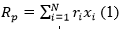2.       Risk of portfolio: Risk of a stock is defined as the tolerance of stock’s return from the mean. The risk of a portfolio is expressed as follows :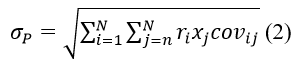Where:

: The covariance between returns of stock i and j expressed as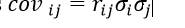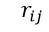:  is the correlation coefficient between i and j.

3.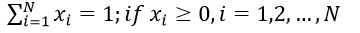Constraints:

Constraints of the attempted model

are as follows: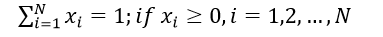It should be noted that the non-negativity of the decision variables is used to prevent short selling.

4.       Proposed Hybrid Genetic Algorithm (HGA):

Portfolio display

In the proposed HGA, any portfolio is represented by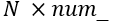bits genes in which N is the number of companies and num_bits is the number of binary bits used for representing the share of each company in the portfolio. The share of company i in the portfolio can be calculated by Solimanpur :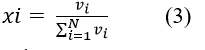Where: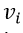: The decimal value of the binary code dedicated for company i.

This formula ensures that, after the execution of genetic operations, the constraints of mathematical models will be satisfied.

Fitness function

Fitness of any chromosome in the proposed HGA is calculated in K directions. Fitness of chromosome S in direction k is computed by Solimanpur :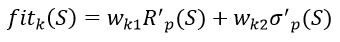Where: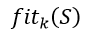: is the fitness value of chromosome S in direction k.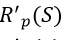: is the normalized value of return of chromosome S.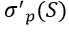: is the normalized value of risk of chromosome S.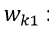is the weight of return in direction k.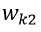: is the weight of risk in direction k.

The normalized values of return and risk of portfolio S in the population are defined as follows Solimanpur : ?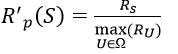(5)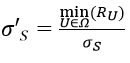(6)

Selection

In the proposed HGA, selection probability of chromosome S in direction k it is proportional to the quality of this chromosome in the direction k, i.e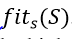. In other words, the higher the fitness of a chromosome, the higher should be selection probability .

### Portfolio Selection via Analytic Hierarchy Process (AHP)

The method proposed an evaluation procedure comprising the following steps :

Step 1: Identify all the criteria to be taken into account in the choice of the portfolio (evaluation) and to build a hierarchy of decision-making.

Step 2: Calculate weights of criteria using AHP method.

These steps are performed in the following subsections.

Evaluation criteria

Selecting the appropriate portfolio of performance measures to provide the necessary information to investors to assess the effectiveness with which they can invest their money is a vital issue. The performance appraisal is primarily related to the determination of how a particular investment portfolio has performed compared to a benchmark comparison. To effectively manage portfolio selection, it is necessary to consider the critical factors that reflect investor behaviour and the state of the financial market. The different stakeholders within a decision process can be relatively diverse, with different objectives and conflicts of value systems [23-29]. In this respect, a key concept is the relationship between risk and return. Each performance index provides a different perspective on the balance between the level of return and the exposure to risk. Therefore, in this study, seven measures, return, risk, beta, liquidity ratio, Sharpe ratio, Treynor’s Ratio (TR) and Jensen’s alpha (Alpha ratio) have been identified as criteria, which affect decision of investors in portfolio selection. Other criteria are discussed in the following subsections.

Beta

Beta measures the price volatility of a share compared to the rest of the market. This measure of risk is defined as :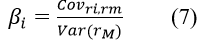Where: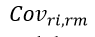: The covariance between the rates of return of shares i and the return of the market or index.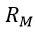: The rate of return of the market portfolio.

Liquidity ratio

This ratio indicates the annual liquidity of a stock. Haugen can then calculate liquidity of a portfolio :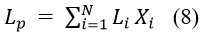Where: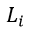: The liquidity of share i.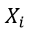: The percentage of share i in the portfolio.

Sharpe ratio

Sharpe ratio or RVAR measures the return into the portfolio risk (standard deviation of return) . It can be defined as this ratio: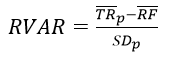(9)

Where: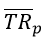: The mean of portfolio return in a certain period.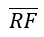: The mean of non-risk return rate in time set.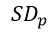: The standard deviation of portfolio return in time set.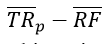: The surplus return of portfolio.

This ratio measures the surplus return of portfolio versus a risk unit. The higher the RVAR of a portfolio, the higher will be its desirability.

Treynor’s ratio (TR)

TR measures the proportion of extra return on beta . Treynor defines this ratio: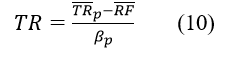Where: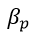: The systematic risk of portfolio (beta ratio).

This ratio measures the excess return of portfolio versus portfolio’s systematic risk unit.

Alpha ratio

Alpha ratio is linked to Treynor’s ratio and, hence, it provides a classification that is close to the performance of a portfolio . This ratio is defined as: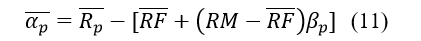The weakness and strength of a portfolio’s performance has two sources: The first is the portfolio manager's ability to select appropriate shares, and the second is their ability to make appropriate decisions over time and to assess threats and market opportunities. Obviously, the manager who considers these aspects will have a better performance in managing the portfolio. The benefit of using this ratio is the possibility to measureandat the same time .

The analytic hierarchy process methodology

AHP includes six basic steps, as summarized in the following [18,22]:

Step 1: AHP breaks down a complex multi-criteria decision-making problem to several small sub-problems each with a single criterion. Thus, the first step is to break the problem of decision in a hierarchy with a goal at the top, criteria and sub-criteria at levels and sub-levels and alternative decisions at the bottom of the hierarchy.

Step 2: The decision matrix, which is based on Saaty’s nine-point scale, is constructed. The decision-maker uses the fundamental scale defined by Saaty to assess the priority score.

Step3: It involves pairwise comparison of the elements of the constructed hierarchy. The aim is to set their relative priorities with respect to each of the elements at the next higher level.

Step 4: AHP also calculates an inconsistency index to reflect the consistency of decision maker’s judgments during the evaluation phase. The inconsistency index in both the decision matrix and in pairwise comparison matrices can be calculated by the following equation :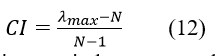The closer the inconsistency index to zero; the greater will be the consistency of decision-maker’s judgments. The consistency of the assessments is ensured if the equality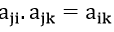holds for all criteria. The relevant index should be lower than 0.10 to accept the AHP results as consistent. If this is not the case, the decision-maker should go back to Steps 2 and 3 and redo the assessments and comparisons.

Step 5: Before any calculation, the comparison matrix has to be normalized. To normalize, each column is divided by the sum of entries of the corresponding column. In that way, a normalized matrix is obtained in which the sum of the elements of each column is 1.

Step 6: The relative values obtained in the third step should satisfy: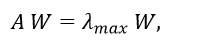(13)

Where:

A: Represents the pairwise comparison matrix.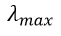: The highest eigenvalue.

W: Weight of the vector or elements.

If there are elements at the higher levels of the hierarchy, the obtained weight vector is multiplied by the weights of the elements at the higher levels, until the top of the hierarchy is reached. The alternative with the highest weight is finally considered as the best alternative.

Questions of the Study

The study questions are:

·         Can HGA and AHP be used in selection of optimum portfolio?

·         Can Efficient Frontier (EF) be identified using HGA?

·         Can AHP select the optimum portfolio among the portfolios obtained by HGA?

The study provides the population, sample, sources of data and variables of the study. Mathematical model and the methodology of the HGA and AHP are also reviewed and in the last hypotheses of the study. The following section presents data analysis, process and implementation of the HGA, AHP and their results.

Data Analysis

This section shows the descriptive statistics of the study variables, and then examines the ability of the HGA and AHP to select the optimum portfolio. In particular this section aims to answer the questions and test the hypotheses of this study.

Hybrid genetic algorithm process and implementation

This section is going to discuss the implementation of the methodology of the HGA. To implement the previously discussed methodology, we created an application using Visual Studio 2010, and C#.NET as a programming language . Visual studio 2010 is an integrated development environment used to create a variety of computer programs, you can select from many available programming languages such as C++,C# ,Visual Basic...etc. This study used C# language, C# is a general-purpose programming language used to create windows forms applications for any purpose. As Figure 1 show, the first step in this work is to read the stocks data. In this step, the program reads the stocks data and creates an object for each stock, and then loads the daily data return for each stock. After that it uses the stocks, data to create 10,000 portfolios, where by each portfolio consists of six stocks. After that it created the portfolios, it uses the daily data return of the assets to calculate the portfolios risk and return. Then it use the portfolios risk and return to evaluate the fitness of the portfolios and select the top 10 portfolios to be used in the HGA. After that, it uses the top 10 portfolios in the crossover process to generate the second generation of portfolios. Finally, it selects the top 10 portfolios from the second-generation portfolios and draws the efficient frontier.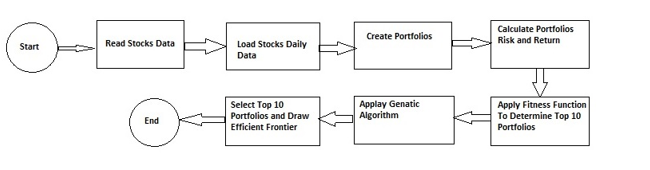Figure 1: Flowchart of the Implementation Process.

### Implementation details

The study gathered the stocks data from Amman Stock Exchange website for the year 2015 and used the data to create excel sheets and notepad files.

As Figure 2 shows, using the file menu and clicking the “Select Data” option, the program will open a select file dialog which allows us to select the stocks data from a notepad (.txt) file.Figure 2: Creating a File and Selecting Data Using C#.

Then the program will read the file data and loads it to the system and create an object for each stock which consists of five characteristics, which are:

1.       Stock ID: unique integer value varies between 60-120 which is used to identify each stock.

2.       Stock Code: Company code as in Amman Stock Exchange.

3.       Return: Stock expected return.

4.       Risk: Stock risk.

5.       Daily Data: The stock daily return.

We have assigned a unique ID for each stock which varies from 60-120. The ID will be used to calculate the weight of each stock in the portfolio, in a manner that the stock with the highest expected return has the highest ID and so on. It would be natural to choose the ID’s from 1-60 but we chose to select the ID’s from 60-120 for the following reason:

In the 1-60 ID’s the share of each stock will dramatically vary between the following values:

·         Highest weight would be the result of company with ID 60 to be assigned in a portfolio with the stocks of ID’s 1,2,3,4 and 5. In this situation the Stock with ID 60 will have a weight of 60/(60+1+2+3+4+5) = 80%.

·         Lowest weight would be the result of company with ID 1 to be assigned in a portfolio with the stocks ID’s 60, 59, 58, 57 and 56. In this situation the Stock with the ID 1 will have a weight of 1/ (60+59 + 58+57+56+ 1)= 0.34%

However, in the situation of ID’s 60-120 we had the weights vary between 9.2% and 27.9%. As we can see, the second approach gives a better chance for the assets to affect the portfolio return and risk, and eliminates the possibility of a single asset to dominate the portfolio. After we have loaded the stocks data, we can load the stocks daily data by clicking the “Load daily data” option as shown. By clicking, the “Load daily data” option the program will show a select file dialog box, which allows us to select notepad file (.txt) which contains each stock and its daily data. Thus, the program will load the daily data for each stock into the stocks objects, which is going to be used in the process of portfolios risk and return calculation [31-36].

Having the stocks are created and the daily data are loaded, we can create the portfolios, by clicking the “Create Portfolios” option shown in Figure 2. The program will use the stocks data to create 10,000 portfolios where each portfolio consists of six different stocks to be selected randomly from the previously created 60 stocks. When the portfolios creation is done, we can calculate the risk and return for each portfolio by clicking the “Portfolio Risk & Return” option from the Calculate menu, as in Figure 3.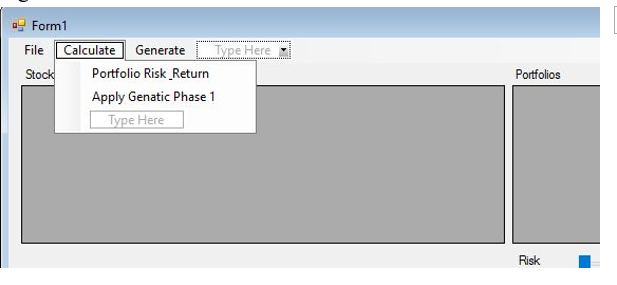Figure 3: Calculate risk and return.

By clicking the “Portfolio Risk and Return” option the program will calculate the risk and return for each portfolio as follows. Portfolio return will be calculated according to the following formula :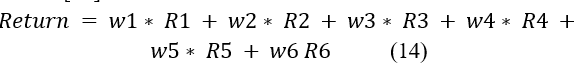Where:

W: The weight of each stock in the portfolio.

R: The rate of return of each stock in the portfolio.

The weight for each stock in the portfolio is calculated by dividing the asset ID over the summation of the total assets IDs of the portfolio.

The 6 asset portfolio risk will be calculated according to the following formula :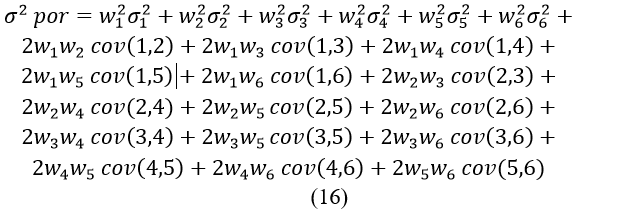And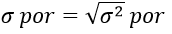To calculate the covariance we used the stocks daily data to calculate the mean and variance for each stock and followed the following formula to calculate the covariance between each two stocks in any portfolio: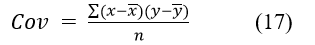Where: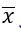and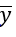: are the sample means return averages.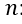: is the sample size.

After we calculate the risk and return for each portfolio, we apply the following fitness function to calculate the fitness factor for each portfolio :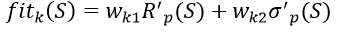(18)

Where: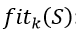: fitness value of chromosome S in direction k.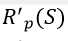: normalized value of return of chromosome.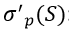: normalized value of risk of chromosome.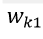: weight of return in direction.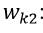weight of risk in direction.

To produce our results we used the following weights for risk and return as shown in (Table 1). After we calculate the fitness value for each portfolio, we select the top 10 portfolios based on the highest fitness value.

Table 1: Fitness function weights.

 Weight of risk Weight of return 50% 50% 40% 60% 80% 20% 20% 80% 60% 40%

Now after we have determined the top 10 portfolios it is the time to use HGA. In the HGA, each portfolio is treated as a gene, where each gene consists of six chromosomes which represent the assets of the portfolio. A chromosome for a portfolio consists of the following assets {114, 120, 86, 74, 62, 91} would be [1110010 1111000 1010110 1001010 0111110 1011011] where each seven digits represent the chromosome for each asset which are the result of the ID conversion from decimal to binary. The purpose of the HGA is to swap chromosomes between the best genes to generate better chromosomes. To do so, we separated the assets of the top 10 portfolios and used these assets to create the second generation of the portfolios using HGA . After we separated the genes from which the chromosomes are created, we used these genes as the basis to create the new portfolios, which is known as the Crossover operation. We have created portfolios six times the number of the successful genes. The created portfolios are referred to as the second-generation portfolios [38-41].

After we created the second-generation portfolios, we selected the top 10 portfolios from the second-generation portfolios and created the efficient frontier graph, as Figure 3.

### Coding Section of Preparing the HGA

So far, we have discussed the workflow of the program in general. In this section, we are going to discuss the work in more technical details and we are going to describe the code we used to create the application. Figure 4 shows the interface of the program we created. As we can see, the interface contains three menus, five grid views and a graph space to draw the efficient frontier and a navigation bar which is used to select the weight of the risk and return that used in the fitness function.Figure 4: Program interface.

The left grid views are used to list the stocks used in creation of the portfolios. Where the right grid views are used to list the portfolios created in the different stages of the program run. The top left grid view is used to list all the available stocks which we created from the data we extracted from Amman Stock Exchange website. The bottom left grid view is used to list the stocks which are used to create the optimum portfolios to be used in the HGA to create the second generation portfolios . The right top grid view is used to display the 10000 portfolios we created using the stocks listed in the top left grid view, where each portfolio consists of six different stocks.

The right middle grid view is used to list the optimum portfolios which consist of the stocks listed in the bottom left grid view. The right bottom grid view is used to list the second generation portfolios which are the result of the genetic algorithm and the crossover operation. As Figure 5 shows we have five different options which work as follows: